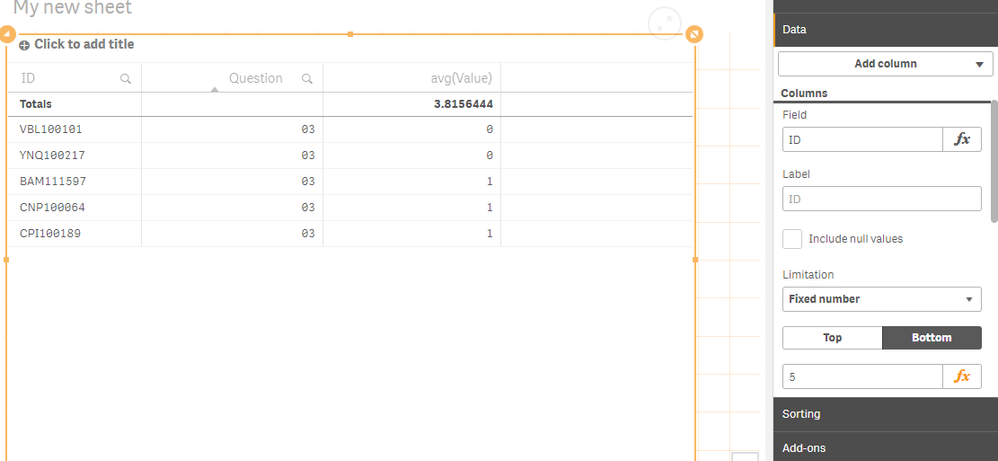# App Development

Announcements
QlikWorld 2023, a live, in-person thrill ride. April 17 - 20, 2023, in Las Vegas! REGISTER TODAY
cancel
Showing results for
Did you mean:Specialist

## Need a help in correcting the set analysis Expression

Hello All,

I am trying to write a set analysis expression to get  Bottom 5 values.

The data has three column, ID,Question,Value.

In a straight table in took

Dimension:Question

Expression:

aggr(avg({<ID={"=Rank(-avg(Value),4)<=5"}>}Value),Questions)

Output: By using above expression the out put isWhich is Wrong ,Let say for Question :03 the Bottom 5 avg value has to be =0.60(0+0+1+1+1/5)but i am getting 1.00(1+1+1+1+1/5) as output same with rest .

what i find out is my expression is ignoring zero value rows and considering only 1 and above values rows .

if i select a value from question let say 03 then i am getting required output which is 0.60 ,without selection i am not gettingSO how to include zero value rows in my expression

PFA sample data

Thanks

because

Labels (1)

10 RepliesMVP

Try this

`Avg(Aggr(If(Rank(-Avg(Value), 4) <= 5, Value), Questions, ID))`Specialist
Author
wat about if i need avg of Bottom 100 values

is this right.
Avg(Aggr(If(Rank(-Avg(Value), 4) <= 100, Value), Questions, ID))MVP
I would think so...Specialist
Author
Some where some thing is missing for bottom 5 i am getting ,but for bottom 100 i am missing few values using above expressionMVP
Can you elaborate with an example or a sample?Specialist
Author
Hi all,
Is there any other way in writing below expression
aggr(avg({<ID={"=Rank(-avg({<FY={\$(vFY)}>}Value),4)<=5"}>}Value),Questions)

Variable
vFY=max(FY)

where FY isa field with value,2018,2017,2016,2015

i tried below expression
Avg(Aggr(If(Rank(-Avg(Value), 4) <= 5, Value), Questions, ID))
but when i put FY in expression
Avg(Aggr(If(Rank(-Avg({<FY={\$(vFY)}>}Value), 4) <= 5, Value), Questions, ID))
i am not getting proper outputMVP
What exactly are you trying to accomplish by re writing your set analysis expression? Is it not working the way you expect or you just want to use another way of doing the same thing?Specialist
Author
HI,
The thing is ,i have got 4 FY years of data ,so in each fiscal year there might be differ in ID's which falls under top 5 ,so i am trying to differentiate ID's w.r.t there Fiscal year .So that is reason why i am trying to put FY in set analysis .
Avg(Aggr(If(Rank(-Avg({<FY={\$(vFY)}>}Value), 4) <= 5, Value), Questions, ID))
Variable
vFY=max(FY)
where FY is a field with values=2018,2017,2016,2015
But the issue is when i have not selected any Fiscal year by default it should pick max FY value which is (2018),but in my case it is not working properly unless i select a Fiscal YearSpecialist
Author

Continue 2.....

Before selecting any value from FYAfter Selecting 2018 from FYWhich is correct output should get same even if i havent select any value from FY field because i am passing Max value through variablePFA the raw data for referenceTags
Community Browser